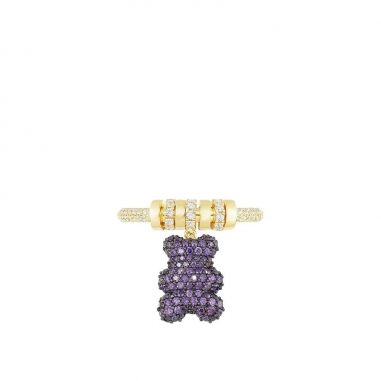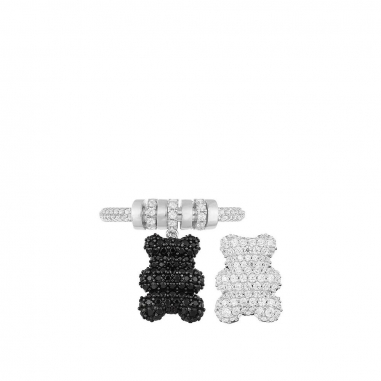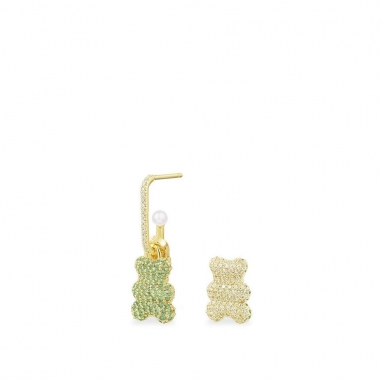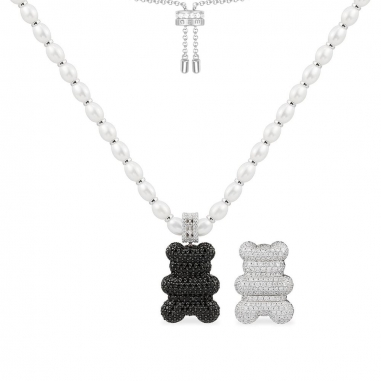227 搜索結果 / ITEMS

# 女仕

#### 搜索過濾

• 保養
• 彩妝
• 香氛
• 女仕
• 男仕
• 數位家電
• 兒童
• 旅行居家
• 伴手禮
• 酒類
• 免稅站獨賣

• 臉部保養
• 身體護理
• 手足護理
• 頭髮護理
• 男仕保養
• 保養特惠組
• 免稅獨賣
• 寶寶護理
• 深刻台灣
• 臉部彩妝
• 眼部彩妝
• 唇部彩妝
• 彩妝特惠組
• 彩妝工具及其他
• 免稅獨賣
• 手足身體彩妝
• 女性香水
• 男性香水
• 中性香水
• 香水特惠組
• 居家香氛
• 免稅獨賣
• 免稅獨賣
• 女用包
• 配件
• 飾品
• 珠寶
• 女錶
• 女裝
• 女鞋
• 旅行用品
• 免稅獨賣
• 男用包
• 配件
• 飾品
• 珠寶
• 男錶
• 男裝
• 男鞋
• 旅行用品
• Apple專區
• 手機與配件
• 電腦與平板
• 相機與攝影
• 遊戲與主機
• 智慧穿戴/耳機音響
• 家電
• 美容健康按摩
• 旅行與外出
• 免稅獨賣
• 免稅獨賣
• 兒童服飾配件
• 兒童清潔保養
• 兒童玩具
• 兒童文具
• 兒童生活用品
• 旅行用品
• 生活用品
• 風格家居
• 玩具玩偶
• 動漫角色
• 台灣特色
• 免稅獨賣
• Hello Kitty 與三麗鷗
• 巧克力與進口食品
• 台式零食點心
• 食材調味料
• 沾拌醬/調理包
• 茶/飲品
• 保健食品
• 免稅獨賣
• 免稅獨賣
• 威士忌
• 白蘭地
• 中式白酒
• 葡萄酒
• 調酒類
• 清酒/梅酒/啤酒
• 白酒/氣泡酒/香檳
• 其他
• 免稅獨家
• 保養
• 男仕保養
• 保養特惠組
• 彩妝
• 香氛
• 女仕
• 男仕

• 臉部清潔
• 導入液
• 化妝水
• 精華液
• 乳液/乳霜/凝膠
• 眼唇護理
• 面膜
• 防曬
• 護理油
• 臉部特殊護理
• 美容用品
• 身體清潔
• 身體乳液/乳霜
• 體香劑
• 美胸
• 身體防曬
• 美體產品
• 身體特殊護理
• 護手霜
• 護足霜
• 頭髮清潔
• 頭髮潤絲
• 頭髮特殊護理
• 美髮造型
• 清潔/刮鬍
• 男仕化妝水
• 男仕精華液
• 男仕乳液/乳霜
• 男仕眼唇護理
• 男仕特殊護理
• 寶寶清潔
• 寶寶乳液/乳霜
• 其他寶寶護理
• 隔離霜/飾底乳
• 粉底
• 粉餅
• 遮瑕
• 蜜粉
• 腮紅/修容打亮
• BB霜/CC霜
• 眉筆/眉粉/染眉膏
• 眼線
• 眼影
• 睫毛膏
• 唇膏
• 唇筆
• 唇釉/唇蜜/唇露
• 指甲油/美甲
• 套組
• 女性Parfum 香精
• 女性E.D.P. 香水
• 女性E.D.T. 淡香水
• 女性Cologne 古龍水
• 女性香水綜合套組
• 其他女性香水
• 男性Parfum 香精
• 男性E.D.P. 香水
• 男性E.D.T. 淡香水
• 男性Cologn 古龍水
• 男性香水綜合套組
• 中性Parfum 香精
• 中性E.D.P. 香水
• 中性E.D.T. 淡香水
• 中性Cologn 古龍水
• 中性香水綜合套組
• 其他中性香水
• 迷你香水組
• 綜合套組
• 後背包
• 肩背包
• 斜背包
• 手提包
• 手拿包
• 托特包
• 腰包
• 化妝包
• 配件包
• 皮夾/零錢包
• 卡夾
• 太陽眼鏡
• 帽子/圍巾
• 皮帶
• 其他配件
• 耳環
• 戒指
• 手鍊手環
• 項鍊與項墜
• 髮飾
• 其他飾品
• 耳環
• 戒指
• 手鍊手環
• 項鍊與項墜
• 金飾
• 精品錶
• 休閒錶
• 兒童錶
• 對錶
• 手錶配件
• 涼鞋/拖鞋
• 休閒鞋/平底鞋
• 運動鞋
• 跟鞋
• 靴子
• 旅行袋
• 旅行箱
• 兒童箱包
• 其他旅行用品
• 後背包
• 肩背包
• 斜背包
• 手提包
• 手拿包
• 托特包
• 腰包
• 配件包
• 皮夾/零錢包
• 卡夾
• 太陽眼鏡
• 帽子/圍巾
• 領帶/袖扣
• 皮帶
• 其他配件
• 耳環
• 戒指
• 手鍊手環
• 項鍊與項墜
• 精品錶
• 休閒錶
• 兒童錶
• 對錶
• 手錶配件
• 涼鞋/拖鞋
• 休閒鞋
• 運動鞋
• 皮鞋
• 靴子
• 旅行袋
• 旅行箱
• 兒童箱包
• 其他旅行用品
• 充電/傳輸
• 手機
• PS5主機/周邊
• Switch主機
• Switch周邊
• Switch遊戲
• 耳機
• 音響
• 智慧穿戴
• 周邊配件
• 吸拖整燙
• 廚房家電
• 影音電視
• 運動用品
• 照明燈飾
• 消毒清淨除溼
• 季節家電
• 理髮電鬍刀
• 潔牙電動牙刷
• 吹整造型
• 健康按摩放鬆
• 網卡/Wifi機
• 行動電源
• 萬國插頭/轉接頭
• 充電線
• 頸枕被毯眼罩
• 行李箱/袋
• 盥洗包/組合
• 護照套/夾
• 其他旅行用品
• 文具用品
• 提袋包包
• 杯盤器皿
• 保溫瓶/水壺
• 其他生活用品
• 木製擺飾
• 琉璃擺飾
• 皮革擺飾
• 銀製擺飾
• 音樂鈴
• 水晶球
• 其他家居擺飾
• 模型
• 樂高
• 玩偶
• 手作益智
• 其他玩具玩偶
• 漫威與DC
• 星際大戰
• Disney系列
• 其他動漫角色
• 清潔護膚
• 衣著飾品
• 吊飾擺設
• 提袋包包
• 其他台灣特色
• Kitty 包包/旅行
• Kitty 餐具水瓶
• Kitty 吊飾玩偶
• Kitty 服飾配件
• Kitty 文具擺件
• Kitty 特殊周邊
• 三麗鷗其他明星
• 茶葉零食點心
• 巧克力
• 餅乾
• 糖果零嘴
• 泡麵
• 餅乾/蛋捲
• 果乾/蜜餞
• 蛋豆/堅果
• 魷魚絲/肉乾
• 糕酥/鳳梨酥
• 糖果/牛軋糖
• 其他零食點心
• 罐頭調料
• 泡麵拌麵
• 米麵/即食包
• 沾拌醬/油醋調料
• 茶葉/茶包
• 咖啡
• 沖泡/調味
• 臉部保養
• 身體護理
• 頭髮護理
• 臉部彩妝
• 唇部彩妝
• 彩妝工具及其他
• 女性香水
• 中性香水
• 男性香水
• 香水特惠組
• 女用包
• 女鞋
• 配件
• 飾品
• 男用包
• 男鞋
• 配件

• 預設
• 時間 (近到遠)
• 價格 (高到低)
• 價格 (低到高)
• 品牌名稱 (A-Z)
• 品牌名稱 (Z-A)

• 顯示 48 樣
• 顯示全部

#### 數量顯示

NT\$ 4,750

NT\$ 5,600

NT\$ 3,350

NT\$ 3,350

NT\$ 5,450

NT\$ 3,250

NT\$ 2,500#多款 APM Monaco YUMMY ULTRA VIOLET BEAR RING YUMMY ULTRA VIOLET BEAR 戒指

NT\$ 5,150#多款 APM Monaco YUMMY BABY MOOD RING YUMMY BABY MOOD BEAR 戒指

NT\$ 5,150

NT\$ 2,100

NT\$ 2,400

NT\$ 6,550

NT\$ 9,250

NT\$ 3,250APM Monaco YUMMY BABY WONDERLAND BEAR EARRING YUMMY BABY WONDERLAND BEAR單只耳環

NT\$ 3,900

NT\$ 7,250

NT\$ 7,250

NT\$ 3,250

NT\$ 4,950

NT\$ 5,450

NT\$ 3,700

NT\$ 8,000

NT\$ 5,450

NT\$ 4,100

NT\$ 3,800

NT\$ 3,250

NT\$ 9,500

NT\$ 8,100APM Monaco YUMMY XL MOOD BEAR NECKLACE YUMMY XL MOOD BEAR 珍珠可調節項鍊

NT\$ 13,050

NT\$ 2,950

NT\$ 5,250

NT\$ 2,500

NT\$ 2,750

NT\$ 5,450

NT\$ 5,500

NT\$ 5,500

NT\$ 22,900

NT\$ 22,900

NT\$ 24,500

NT\$ 26,500

NT\$ 31,500

NT\$ 15,900 NT\$ 11,130

NT\$ 13,900 NT\$ 9,730

NT\$ 24500 NT\$ 12,250

NT\$ 27500 NT\$ 13,750

NT\$ 22900 NT\$ 16,030